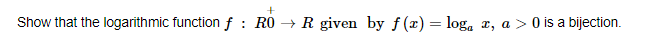# Show that the logarithmic function f :

Question:Solution:

$f: R^{+} \rightarrow R$ given by $f(x)=\log _{a} x, a>0$

Injectivity:
Let x and y be any two elements in the domain (N), such that f(x= f(y).

$f(x)=f(y)$

$\log _{a} x=\log _{a} y$

$\Rightarrow x=y$

Surjectivity:
Let y be any element in the co-domain (R), such that f(x) = y for some element x in R+ (domain).

$f(x)=y$

$\log _{a} x=y$

$\Rightarrow x=a^{y} \in R^{+}$

So, for every element in the co-domain, there exists some pre-image in the domain.

$\Rightarrow f$ is onto.

Since $f$ is one-one and onto, it is a bijection.